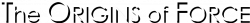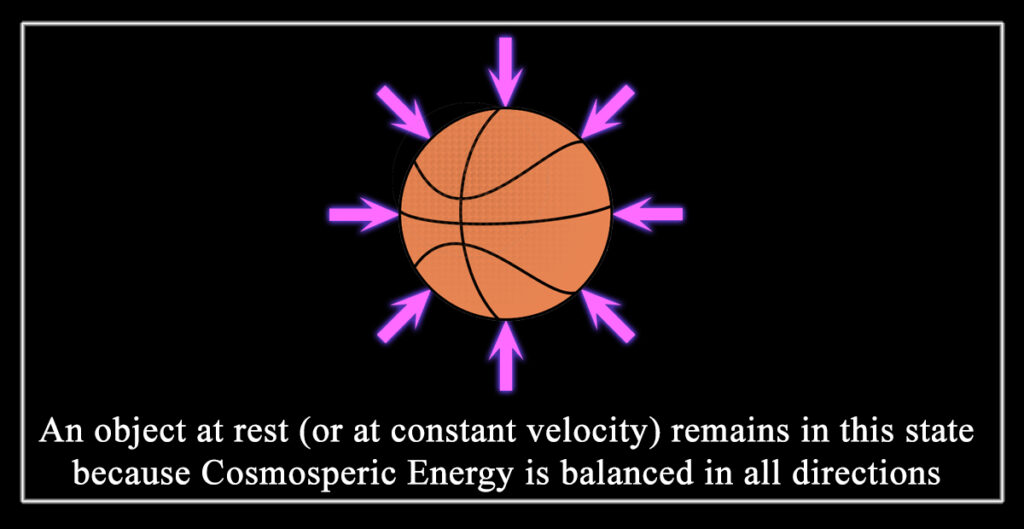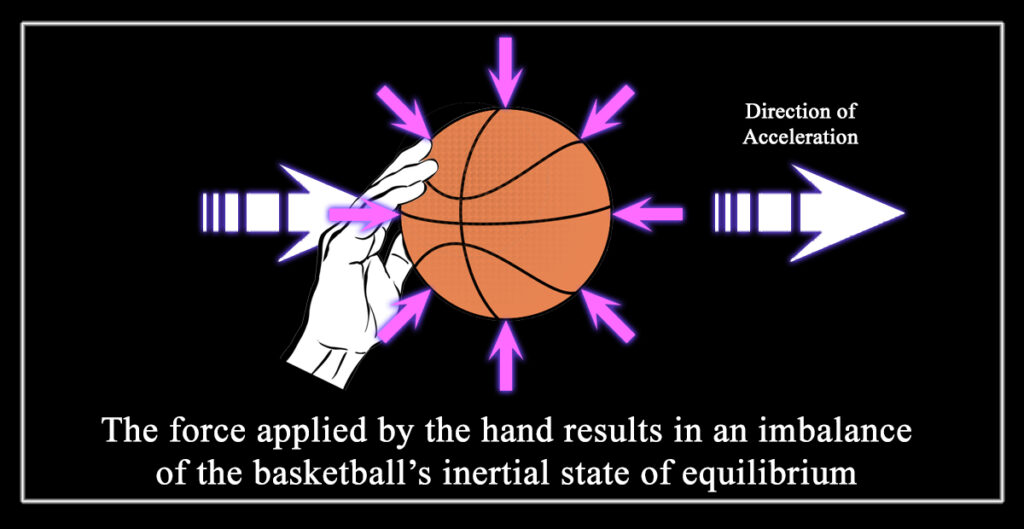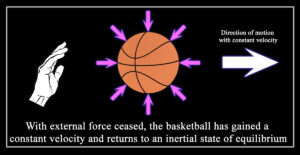## Part II - Inertia

Inertia is the tendency of an object to remain at rest or in motion. Newton’s First Law of Motion states that an “object will remain at rest or move at a constant speed in a straight line unless it is acted on by an unbalanced force.”

What Newton didn’t state, and what he couldn’t possibly explain within his model of the universe, was why objects remain at rest or move at a constant speed.

When a transmission of Cosmospheric Energy encounters a particle of matter along its travels, what occurs depends on two things: 1) the type of energy and its angle of the approach, and 2) the type and state of the matter at the moment of the encounter.

In some cases, Cosmospheric Energy passes by or through the matter and both are unaffected. The rest of the time, the energy is absorbed, deflected, or reflected by the matter. In these cases, the energy exerts a momentary influence upon the matter and vice-versa. We call this influence force, and that force causes an acceleration of the matter which creates changing motion.

Whether an object is at rest, or at a constant velocity, the Cosmosphere exerts relatively equal amounts of energy upon the object from all sides. The state of inertia is a state of equilibrium with Cosmospheric Energy bombardment.

In order to budge an object out of an inertial state, an external force must be applied to overcome the Cosmospheric Energy exerted upon the opposing side.

An external force applied to one side of an object throws off the balance of Cosmospheric forces that were holding it in inertial equilibrium. The resulting uneven application of forces cause an acceleration in the direction of the force applied.

For example, imagine a basketball floating in outer space, completely motionless and remaining at rest. Cosmospheric Energy is striking its surface equally in all directions, creating forces in all directions inwards and keeping the ball motionless. In order to get that ball to move, we have to push it.In an inertial state, the forces affecting any given side of the basketball are negated and cancelled out by the equal forces pressing on the opposing side. (Assuming of course that our basketball is located near the effective center of the universe – see Part VIII). Only with an external force can we upset the balancing Cosmospheric Energy forces holding the basketball in a state of inertial equilibrium.If we are successful in applying enough force against one side of the ball to overcome the Cosmospheric Energy forces on the opposing side, we achieve acceleration in the ball which will continue to increase its velocity until we stop applying the external force.

Once all external forces are withdrawn, it regains equilibrium with Cosmospheric Energy forces applied from all directions. It therefore regains an inertial state and will continue travelling with unchanged constant velocity indefinitely.Newton’s First Law could be more clearly stated as “an object will remain at rest or move at a constant speed in a straight line unless it is acted on by an unbalanced force, because an unbalanced force must be used to overcome the Cosmospheric Energy forces keeping the object in equilibrium.”

In reality, almost everything in the universe is in a state of acceleration. Absolute inertia only exists in one place – the effective dead center of the Cosmosphere. This likely is not exactly the geographic dead center of the universe, but rather a point somewhat nearby the center in which all Cosmospheric Energy bombardment from all directions is perfectly equal. Whether or not an object is actually trapped and held there at this moment, it would be a very interesting place to visit.

In our own neck of the woods, likely quite far from the center of the universe, everything nearby has (more-or-less) an equal amount of Cosmospheric imbalance, meaning relative inertia. The concepts of absolute and relative inertia will be elaborated further in Part VII which explains the expanding and accelerating universe.

### Prev

Current Chapter: Part II
Inertia

### Previous Chapter

Current Chapter:
Part II – Inertia